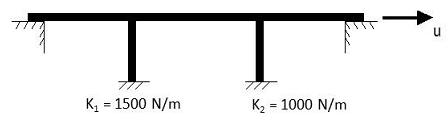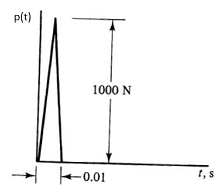### What is the maximum displacement of the bridge deck

Assignment Help Physics
##### Reference no: EM13512

The longitudinal vibrations of a bridge are to be modeled as a single-degree-of-freedom system. The mass of the bridge is 2.5kg.  The stiffnesses of the columns are 1500 N/m and 1000 N/m.  c=10 N-s/m.What is the maximum displacement of the bridge deck?

### Write a Review

#### What is the electric field at the location

Question: Field and force with three charges? What is the electric field at the location of Q1, due to  Q 2 ?

#### Find the magnitude of the resulting magnetic field

A sphere of radius R is uniformly charged to a total charge of Q. It is made to spin about an axis that passes through its center with an angular speed ω. Find the magnitude of the resulting magnetic field at the center of the sphere.

#### Find the equivalent resistance

A resistor is in the shape of a cube, with each side of resistance  R . Find the equivalent resistance between any two of its adjacent corners.